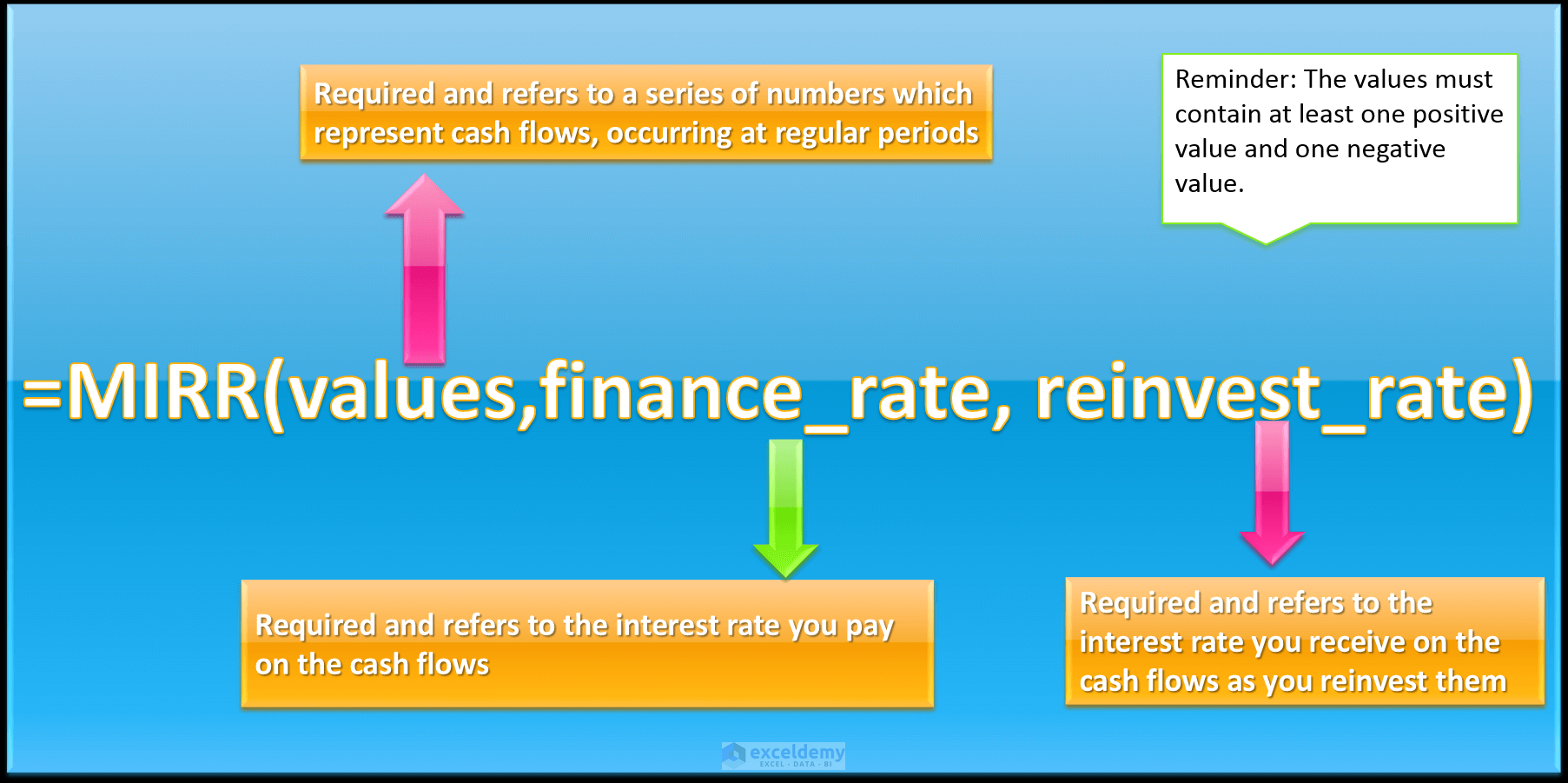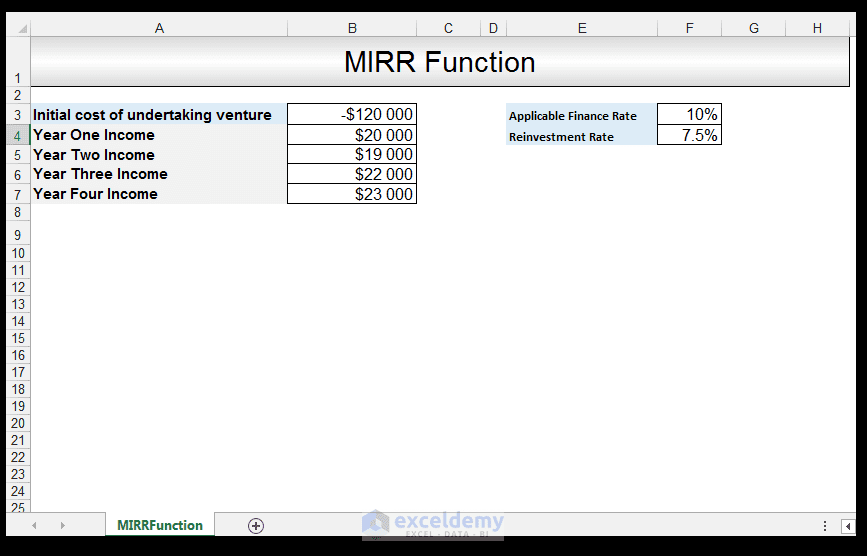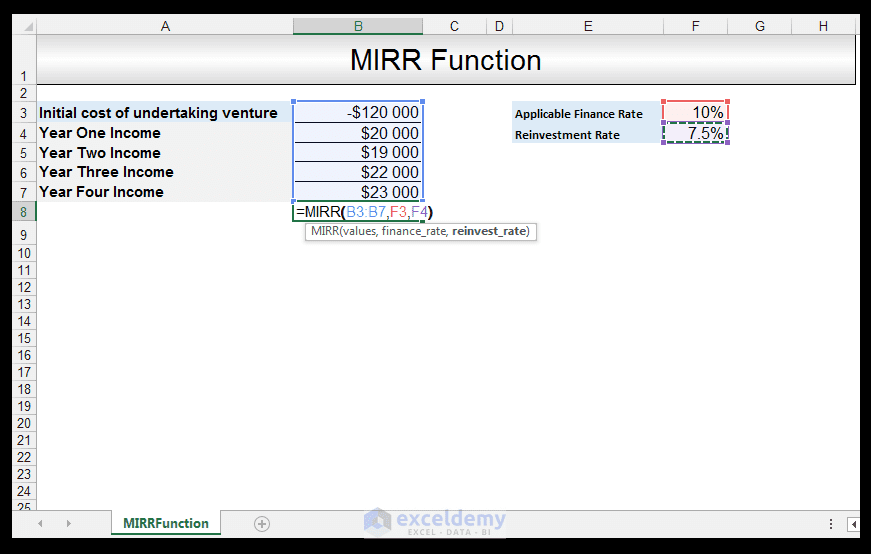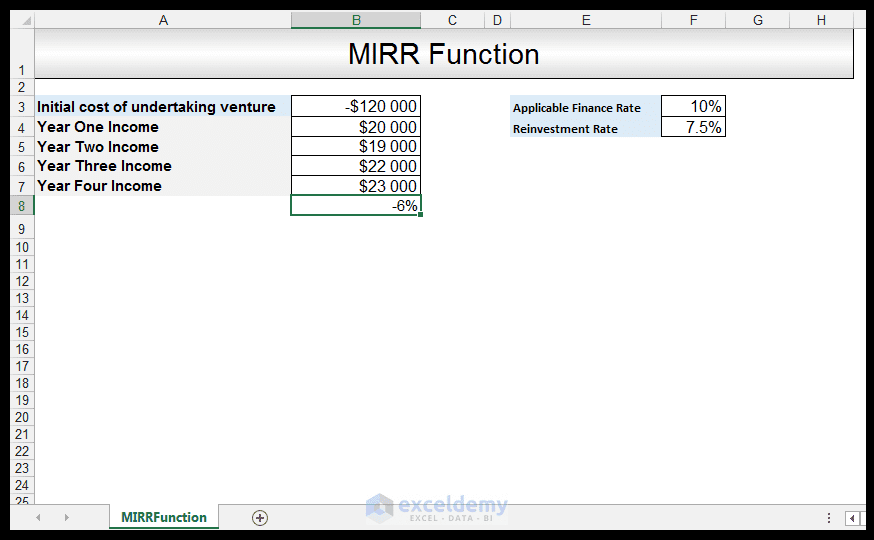Disclosure: This post may contain affiliate links, meaning when you click the links and make a purchase, we receive a commission.

# How to Use the Excel MIRR Function

We have already seen how to use the IRR (Internal Rate of Return) Function in a previous tutorial. The IRR Function is used to calculate the internal rate of return for a series, of given periodic cash flows. The internal rate of return is utilized, in order to give an indication of the potential profitability of an investment/project.  The MIRR (Modified Internal Rate of Return) Function is also utilized to give an indication of the potential profitability of an investment/project.

However, the modified internal rate of return takes into account the initial cost of the investment, as well as the interest received on reinvestment of the cash flows. There are two main problems, with the internal rate of return that the modified internal rate of return, deals with. The first main problem is that the internal rate of return calculation can yield multiple solutions for the same project, which makes comparative analysis difficult at times. The second main problem with the internal rate of return is that it assumes that positive cash flows are reinvested at the same rate of return as the project that generated them when in practice this is rarely the case. In practice, it is more likely that they will be reinvested at a rate that is close to the firm’s cost of capital.

The syntax for the MIRR Function is:So, let’s get started with a simple example to demonstrate when to use the MIRR Function.

Read More: How to Use Compound Interest Formula in Excel

## Calculating MIRR

A quantity surveyor working for a construction company wants to calculate the MIRR for a construction project the company has received. The applicable financing rate is 10%, due to the economic climate, a lower reinvestment rate of 7.5% was reinvested. The initial cost of the undertaking was \$120 000. The income for year one was \$20, 000, for year two \$19, 000, for year three \$22, 000 and for year four, \$23, 000.1) In order to calculate the MIRR, in Cell B8 we input the following formula:

=MIRR (B3: B7, F3, F4)2) Upon pressing CTRL-ENTER, a value of -6 % is returned.The MIRR of a potential project can be utilized, in order to ascertain the potential profitability of an investment or project. In general projects with a MIRR that is greater than the project’s hurdle rate should be accepted. In this case for the period given, the project’s profitability as indicated by the MIRR is not that attractive.

## Conclusion

The MIRR Function handles the shortcomings of the IRR Function and provides a more realistic view of the profitability, of a potential investment or loan. Generally speaking, the IRR can sometimes yield an overly optimistic view of a certain project or investment, therefore it is always worthwhile to calculate the MIRR in order to double check.

The MIRR is also used to compare investments of unequal size, generally speaking, a project with a higher MIRR is more attractive than one with a lower MIRR

Please feel free to comment and tell us what projects/investments, you use MIRR and IRR for in your financial spreadsheets.

IRR versus XIRR Excel Functions [Tutorial]

NPV versus XNPV Excel Functions Tutorial

Calculating the Rate an Investment Achieves Using the RRI Function

How to Calculate Variance in Excel

MIRRFunction

Taryn is a Microsoft Certified Professional, who has used Office Applications such as Excel and Access extensively, in her interdisciplinary academic career and work experience. She has a background in biochemistry, Geographical Information Systems (GIS) and biofuels. She enjoys showcasing the functionality of Excel in various disciplines.

1. Reply